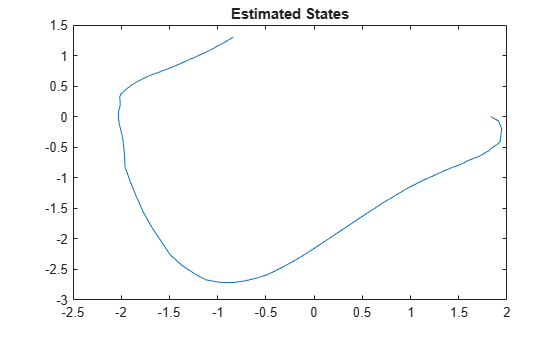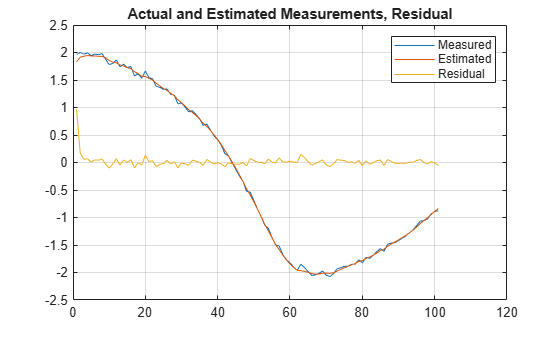# residual

Return measurement residual and residual covariance when using extended or unscented Kalman filter

## Syntax

``````[Residual,ResidualCovariance] = residual(obj,y)``````
``````[Residual,ResidualCovariance] = residual(obj,y,Um1,...,Umn)``````

## Description

The `residual` command returns the difference between the actual and predicted measurements for `extendedKalmanFilter` and `unscentedKalmanFilter` objects. Viewing the residual provides a way for you to validate the performance of the filter. Residuals, also known as innovations, quantify the prediction error and drive the correction step in the extended and unscented Kalman filter update sequence. When using `correct` and `predict` (System Identification Toolbox) to update the estimated Kalman filter state, use the `residual` command immediately before using the `correct` command.

example

``````[Residual,ResidualCovariance] = residual(obj,y)``` returns the residual `Residual` between a measurement `y` and a predicted measurement produced by the Kalman filter `obj`. The function also returns the covariance of the residual `ResidualCovariance`.You create `obj` using the `extendedKalmanFilter` or `unscentedKalmanFilter` commands. You specify the state transition function f and measurement function h of your nonlinear system in `obj`. The `State` property of the object stores the latest estimated state value. At each time step, you use `correct` and `predict` together to update the state x. The residual s is the difference between the actual and predicted measurements for the time step, and is expressed as s = y - h(x). The covariance of the residual S is the sum R + RP, where R is the measurement noise matrix set by the `MeasurementNoise` property of the filter and RP is the state covariance matrix projected onto the measurement space.Use this syntax if the measurement function h that you specified in `obj.MeasurementFcn` has one of the following forms: `y(k) = h(x(k))` for additive measurement noise`y(k) = h(x(k),v(k))` for nonadditive measurement noise Here, `y(k)`, `x(k)`, and `v(k)` are the measured output, states, and measurement noise of the system at time step `k`. The only inputs to h are the states and measurement noise.```

example

``````[Residual,ResidualCovariance] = residual(obj,y,Um1,...,Umn)``` specifies additional input arguments if the measurement function of the system requires these inputs. You can specify multiple arguments.Use this syntax if the measurement function h has one of the following forms: `y(k) = h(x(k),Um1,...,Umn)` for additive measurement noise`y(k) = h(x(k),v(k),Um1,...,Umn)` for nonadditive measurement noise ```

## Examples

collapse all

Estimate the states of a van der Pol oscillator using an extended Kalman filter algorithm and measured output data. The oscillator has two states and one output.

Create an extended Kalman filter object for the oscillator. Use previously written and saved state transition and measurement functions, `vdpStateFcn.m` and `vdpMeasurementFcn.m`. These functions describe a discrete-approximation to a van der Pol oscillator with the nonlinearity parameter $\mathrm{mu}$ equal to 1. The functions assume additive process and measurement noise in the system. Specify the initial state values for the two states as [1;0]. This is the guess for the state value at initial time `k`, based on knowledge of system outputs until time `k-1`, $\underset{}{\overset{ˆ}{x}}\left[k|k-1\right]$.

`obj = extendedKalmanFilter(@vdpStateFcn,@vdpMeasurementFcn,[1;0]);`

Load the measured output data `y` from the oscillator. In this example, use simulated static data for illustration. The data is stored in the `vdp_data.mat` file.

`load vdp_data.mat y`

Specify the process noise and measurement noise covariances of the oscillator.

```obj.ProcessNoise = 0.01; obj.MeasurementNoise = 0.16;```

Initialize arrays to capture results of the estimation.

```residBuf = []; xcorBuf = []; xpredBuf = [];```

Implement the extended Kalman filter algorithm to estimate the states of the oscillator by using the `correct` and `predict` commands. You first correct $\underset{}{\overset{ˆ}{x}}\left[k|k-1\right]$ using measurements at time `k` to get $\underset{}{\overset{ˆ}{x}}\left[k|k\right]$. Then, you predict the state value at the next time step $\underset{}{\overset{ˆ}{x}}\left[k+1|k\right]$ using $\underset{}{\overset{ˆ}{x}}\left[k|k\right]$, the state estimate at time step `k` that is estimated using measurements until time `k`.

To simulate real-time data measurements, use the measured data one time step at a time. Compute the residual between the predicted and actual measurement to assess how well the filter is performing and converging. Computing the residual is an optional step. When you use `residual`, place the command immediately before the `correct` command. If the prediction matches the measurement, the residual is zero.

After you perform the real-time commands for the time step, buffer the results so that you can plot them after the run is complete.

```for k = 1:size(y) [Residual,ResidualCovariance] = residual(obj,y(k)); [CorrectedState,CorrectedStateCovariance] = correct(obj,y(k)); [PredictedState,PredictedStateCovariance] = predict(obj); residBuf(k,:) = Residual; xcorBuf(k,:) = CorrectedState'; xpredBuf(k,:) = PredictedState'; end```

When you use the `correct` command, `obj.State` and `obj.StateCovariance` are updated with the corrected state and state estimation error covariance values for time step `k`, `CorrectedState` and `CorrectedStateCovariance`. When you use the `predict` command, `obj.State` and `obj.StateCovariance` are updated with the predicted values for time step `k+1`, `PredictedState` and `PredictedStateCovariance`. When you use the `residual` command, you do not modify any `obj` properties.

In this example, you used `correct` before `predict` because the initial state value was $\underset{}{\overset{ˆ}{x}}\left[k|k-1\right]$, a guess for the state value at initial time `k` based on system outputs until time `k-1`. If your initial state value is $\underset{}{\overset{ˆ}{x}}\left[k-1|k-1\right]$, the value at previous time `k-1` based on measurements until `k-1`, then use the `predict` command first. For more information about the order of using `predict` and `correct`, see Using predict and correct Commands.

Plot the estimated states, using the postcorrection values.

```plot(xcorBuf(:,1), xcorBuf(:,2)) title('Estimated States')```Plot the actual measurement, the corrected estimated measurement, and the residual. For the measurement function in `vdpMeasurementFcn`, the measurement is the first state.

```M = [y,xcorBuf(:,1),residBuf]; plot(M) grid on title('Actual and Estimated Measurements, Residual') legend('Measured','Estimated','Residual')```The estimate tracks the measurement closely. After the initial transient, the residual remains relatively small throughout the run.

Consider a nonlinear system with input `u` whose state `x` and measurement `y` evolve according to the following state transition and measurement equations:

`$x\left[k\right]=\sqrt{x\left[k-1\right]+u\left[k-1\right]}+w\left[k-1\right]$`

`$y\left[k\right]=x\left[k\right]+2*u\left[k\right]+v\left[k{\right]}^{2}$`

The process noise `w` of the system is additive while the measurement noise `v` is nonadditive.

Create the state transition function and measurement function for the system. Specify the functions with an additional input `u`.

```f = @(x,u)(sqrt(x+u)); h = @(x,v,u)(x+2*u+v^2);```

`f` and `h` are function handles to the anonymous functions that store the state transition and measurement functions, respectively. In the measurement function, because the measurement noise is nonadditive, `v` is also specified as an input. Note that `v` is specified as an input before the additional input `u`.

Create an extended Kalman filter object for estimating the state of the nonlinear system using the specified functions. Specify the initial value of the state as 1 and the measurement noise as nonadditive.

`obj = extendedKalmanFilter(f,h,1,'HasAdditiveMeasurementNoise',false);`

Specify the measurement noise covariance.

`obj.MeasurementNoise = 0.01;`

You can now estimate the state of the system using the `predict` and `correct` commands. You pass the values of `u` to `predict` and `correct`, which in turn pass them to the state transition and measurement functions, respectively.

Correct the state estimate with measurement `y`[`k`]=`0.8` and input `u`[`k`]=`0.2` at time step `k`.

`correct(obj,0.8,0.2)`

Predict the state at the next time step, given `u`[`k`]=`0.2`.

`predict(obj,0.2)`

Retrieve the error, or residual, between the prediction and the measurement.

`[Residual, ResidualCovariance] = residual(obj,0.8,0.2);`

## Input Arguments

collapse all

Extended or unscented Kalman filter, created using one of the following commands:

Measured system output at the current time step, specified as an N-element vector, where N is the number of measurements.

Additional input arguments to the measurement function of the system, specified as input arguments of any type. The measurement function h is specified in the `MeasurementFcn` or `MeasurementLikelihoodFcn` property of `obj`. If the function requires input arguments in addition to the state and measurement noise values, you specify these inputs in the `residual` command syntax. The `residual` command passes these inputs to the measurement or the measurement likelihood function to calculate estimated outputs. You can specify multiple arguments.

For instance, suppose that your measurement or measurement likelihood function calculates the estimated system output `y` using system inputs `u` and current time `k`, in addition to the state `x`. The Um1 and Um2 terms are therefore `u(k)` and `k`. These inputs result in the estimated output

`y(k) = h(x(k),u(k),k)`

Before you perform online state estimation correction at time step `k`, specify these additional inputs in the `residual` command syntax:

`[Residual,ResidualCovariance] = residual(obj,y,u(k),k);`

For an example showing how to use additional input arguments, see Specify State Transition and Measurement Functions with Additional Inputs.

## Output Arguments

collapse all

Residual between current and predicted measurement, returned as a:

• Scalar for a single-output system

• Vector of size N for a multiple-output system, where N is the number of measured outputs

Residual covariance, returned as an N-by-N matrix where N is the number of measured outputs.

Introduced in R2019b